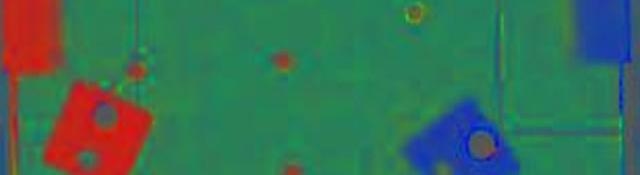## What is normalized RGB?

At times, you want to get rid of distortions caused by lights and shadows in an image. Normalizing the RGB values of an image can at times be a simple and effective way of achieving this.

When normalizing the RGB values of an image, you divide each pixel's value by the sum of the pixel's value over all channels. So if you have a pixel with intensitied R, G, and B in the respective channels... its normalized values will be R/S, G/S and B/S (where, S=R+G+B).

Here's an example (I tried this out when working on the soccer bot I made):

The upper image is the original shot taken from a camera. The lower image is its normalized version. It might not look pretty, but take note of some key changes in the image:

• The shadows are the white edges have vanished
• The black and white circles have become indistinguishable
• The entire goal posts are not one solid colour.

Now this might not be the best picture to showoff normalized RGB. But it gets the point through. (If you find a better example, please let me know!!).

## Writing code for this

We'll write a short function that will convert a given RGB image into a normalized RGB image. We begin by writing the function definition:

```IplImage* NormalizeImage(IplImage* theimg)
{
```

Now we create 3 more images. Each to hold the 3 channels of theimg.

```    IplImage* redchannel = cvCreateImage(cvGetSize(theimg), 8, 1);
IplImage* greenchannel = cvCreateImage(cvGetSize(theimg), 8, 1);
IplImage* bluechannel = cvCreateImage(cvGetSize(theimg), 8, 1);
```

And then, we create 4 images: one to hold the final normalized image and 3 to temporarily hold the channels of the normalized image:

```    IplImage* redavg = cvCreateImage(cvGetSize(theimg), 8, 1);
IplImage* greenavg = cvCreateImage(cvGetSize(theimg), 8, 1);

IplImage* blueavg= cvCreateImage(cvGetSize(theimg), 8, 1);

IplImage* imgavg = cvCreateImage(cvGetSize(theimg), 8, 3);
```

Next, we copy the 3 channels of the original image into redchannel, greenchannel and bluechannel.

```    cvSplit(theimg, bluechannel, greenchannel, redchannel, NULL);
```

Next, we setup loops to iterate through each pixel of the image...

```    for(int x=0;x<theimg->width;x++)
{
for(int y=0;y<theimg->height;y++)
{
```

...and find out the R, G and B values at this pixel:

```            int redValue = cvGetReal2D(redchannel, y, x);
int greenValue = cvGetReal2D(greenchannel, y, x);
int blueValue = cvGetReal2D(bluechannel, y, x);
```

Then, we calculate S = R+G+B:

```            double sum = redValue+greenValue+blueValue;
```

And finally, we set the normalized values into the 3 channel images we created for the final normalized image:

```            cvSetReal2D(redavg, y, x, redValue/sum*255);
cvSetReal2D(greenavg, y, x, greenValue/sum*255);
cvSetReal2D(blueavg, y, x, blueValue/sum*255);
}
}
```

After all this looping, we'll have the final channels of the normalized image. We merge these into the final image:

```    cvMerge(blueavg, greenavg, redavg, NULL, imgavg);
```

Once all of this is done, we erase the temporary images we had created, and return the result:

```    cvReleaseImage(&redchannel);
cvReleaseImage(&greenchannel);
cvReleaseImage(&bluechannel);
cvReleaseImage(&redavg);
cvReleaseImage(&greenavg);

cvReleaseImage(&blueavg);

return imgavg;

}
```

Using the function we just created is simple. You give it an image in the RGB colour space. And it returns a new image in the normalized RGB form.

## You're losing information

The normalized image can be represented using only 2 bytes per pixel (as opposed to 3 bytes per pixel in RGB). Here's how: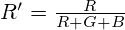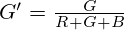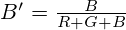But you can represent B' like this as well: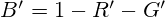So ultimately, all you need to store is the value of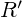and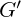. You can calculate the B' component from the other two. Physically, its this loss of information that "removes" the lighting information from the image.

## Conclusion

Hopefully this article helped add a new tool to your arsenal of techniques and algorithms.

#### Related posts#### 7 unique neural network architectures#### All about biological neurons

Utkarsh Sinha created AI Shack in 2010 and has since been working on computer vision and related fields. He is currently at Microsoft working on computer vision.